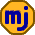## Tuesday, September 29, 2009

### Choosing a Mortgage Term

Choosing a mortgage is a high stakes game. Most people understand the importance of getting a low interest rate, but trying to choose among variable-rate mortgages and fixed-rate mortgages of various durations can be difficult.

A number of factors go into making the best choice of mortgage, and one of those factors is the interest rate offered for the different terms of fixed-rate mortgages. As an example, let’s use the best interest rates at the time of writing on fixed-rate mortgages available from one mortgage broker, Invis, which are as follows:

1 year: 2.55%
2 years: 2.89%
3 years: 3.39%
4 years: 3.74%
5 years: 4.09%
7 years: 5.05%
10 years: 5.40%

If you’re already thinking of taking a 1-year term, you may wonder if you would be better off taking a 2-year term for only an extra 0.34% each year. To compare these choices, it is better to think of the 2-year mortgage as having a rate of 2.55% in the first year and 3.23% in the second year. This second year rate is calculated so that the two years compound out to the 2-year average rate of 2.89%.

So now it’s a little easier to make a choice. If you’re already planning to pay 2.55% in the first year, are you willing to lock in a rate of 3.23% for the second year? If not, stick to the 1-year mortgage.

This reasoning can be extended across all of the terms to produce the following table of rates for each year of a 10-year mortgage:

Year 1: 2.55%
Year 2: 3.23%
Year 3: 4.40%
Year 4: 4.80%
Year 5: 5.50%
Year 6: 7.49%
Year 7: 7.49%
Year 8: 6.22%
Year 9: 6.22%
Year 10: 6.22%

You would use this table as follows. To choose between a 2 or 3-year mortgage, you have to decide whether you’re willing to lock in a rate of 4.40% in the third year. To choose between a 5 and 7-year mortgage, you have to decide whether you’re willing to lock in a rate of 7.49% in years 6 and 7.

This is the way I looked at things when I chose my first mortgage. I found it to be the only way to make sense of all the choices.

For the Math Geeks:

For those interested in how I came up with the table, here is an example for year 4. If the lender’s interest rates for 3 years and 4 years are r3 and r4, then rate for year 4 in my table (call it R4) satisfies the following rule:

(1+R4)*(1+r3)^3 = (1+r4)^4

This can be solved as

R4 = ((1+r4)^4)/( (1+r3)^3) – 1

The cases where the gap between mortgage terms is longer than one year are a little more complicated. For the case of table entries for years 6 and 7 (R6 and R7), we use the 5-year and 7-year lender rates (r5 and r7) as follows:

(1+R6)*(1+R7)*(1+r5)^5 = (1+r7)^7

Arbitrarily setting R6=R7 allows us to solve this for the year 6 and year 7 rates.

1.2.